## Solution to 1986 Problem 2

 We apply Gauss's Law in integral form: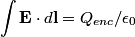\begin{align*}\int \mathbf{E} \cdot d \mathbf{l} = Q_{enc}/\epsilon_0\end{align*} We take the closed surface to be a sphere of radius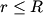$r \leq R$ concentric with the sphere in the problem statement. Also, let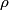$\rho$ be the charge density, which we know is a constant. Then by Gauss's Law, we have,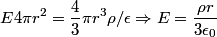\begin{align*}E 4 \pi r^2 = \frac{4}{3} \pi r^3 \rho/ \epsilon \Rightarrow E = \frac{\rho r}{3 \epsilon_0}\end{align*}Thus, we see that the relationship between radius and electric field is a linear one, and so curve C must be correct. Hence the answer is (C). For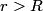$r > R$, the electric field falls off like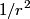$1/r^2$ and is identical to the electric field produced by a point charge with charge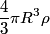$\frac{4}{3} \pi R^3 \rho$.